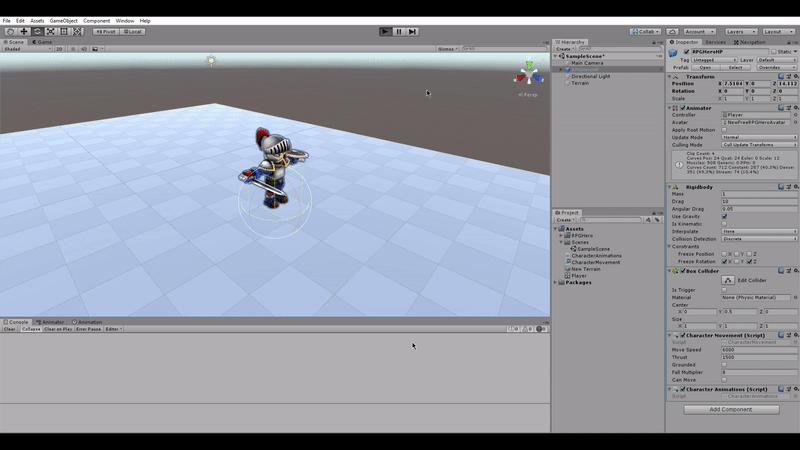# Character faces wrong way for one frame only. Why?

Hi,

When landing from a jump and then moving immediately, my player’s character model will rotate the wrong way for exactly one frame.
The transform of the player GameObject does not change at all.

Any ideas what could be causing this?

I’ll put the code below, sorry if it’s long. But I’m not exactly sure what part is causing this.

``````using System.Collections;
using System.Collections.Generic;
using UnityEngine;

public class CharacterMovement : MonoBehaviour
{

private Camera cam;
public float moveSpeed = 10f;
public float thrust = 4000f;
Rigidbody rb;
[HideInInspector] public Vector3 desiredMoveDirection;
public bool grounded;
public float fallMultiplier = 4f;
Quaternion InAirRotation;
public float downForce = 100f;

public bool canMove;

// Start is called before the first frame update
void Start()
{
cam = Camera.main;
rb = GetComponent<Rigidbody>();
grounded = true;
canMove = true;
}

// Update is called once per frame
void Update()
{

}

void FixedUpdate()
{
if(canMove)//Movement Enabled
{

float horizontalAxis = Input.GetAxis("Horizontal");
float verticalAxis = Input.GetAxis("Vertical");

var forward = cam.transform.forward;
var right = cam.transform.right;

forward.y = 0f;
right.y = 0f;
forward.Normalize();
right.Normalize();

desiredMoveDirection = forward * verticalAxis + right * horizontalAxis;

//Add force to Rigidbody depending on the input.

if(grounded)
{
//Always set the Y axis to 0. - This stops the character trying to look at itself.
Vector3 vel = rb.velocity;
vel.y = 0f;
rb.velocity = vel;

//Rotate to movement only when moving. When Idle, facing wherever player was walking.
if(desiredMoveDirection != Vector3.zero)
{
//Make player look at the direction they're moving.
transform.rotation = Quaternion.Lerp(transform.rotation,Quaternion.LookRotation(rb.velocity),10f);

}
} else
{
//If not grounded but input is detected.
if(desiredMoveDirection != Vector3.zero)
{
//Moving but not Grounded

//Look at direction of movement.
var newRotation = Quaternion.LookRotation(rb.velocity);
//Ignore the movement of the X and Z axis.
newRotation.x = 0;
newRotation.z = 0;
transform.rotation = Quaternion.Lerp(transform.rotation, newRotation, 10f);
}
}

}

//Actions - jumping, attacking, etc.

if(Input.GetButton("Jump"))
{
//Jump
if(grounded)
{
changeSpeed();
grounded = false;
}
}

if(rb.velocity.y <= 0)
{
//If the player is is the air - fall faster.
//rb.velocity += Vector3.up * Physics.gravity.y * (fallMultiplier -1) * Time.deltaTime;
}

if(rb.velocity.y < 0)
{
}
}

private void OnCollisionEnter(Collision other)
{
if(other.gameObject.tag == "Ground")
{
grounded = true;
moveSpeed = 6000f;
}	else { grounded = false; }
}

void changeSpeed()
{
moveSpeed = moveSpeed / 2;
}
}
``````The transform of the player GameObject does not change at all.

If you look at your GIF, you’ll notice that in between most frames, the transform inspector doesn’t update. I think this might mean that the issue does in fact lie in a bad rotation calculation.

I think the culprit is on line 68, where you write `Quaterion.LookRotation(rb.velocity)`. When the character hits the ground, it’s possible that its velocity becomes zero. According to the Quaternion documentation, if the given vector is zero, it returns the identity quaternion (i.e. “no rotation”). Setting this as the value for `transform.rotation` makes your character face the default rotation momentarily, until enough force is applied by line 54 to make rb.velocity nonzero.

I can see a couple of other potential problems:

On line 83, you write `transform.rotation = Quaternion.Lerp(transform.rotation, newRotation, 10f);` Passing 10f as the interpolation parameter makes this equivalent to `transform.rotation = newRotation` (see here). You do this on line 68 also.

On lines 79-82, instead of setting the x and z fields of newRotation to 0, you should instead set the y field of the look direction (rb.velocity) to 0. So something like this:

`var lookDirection = rb.velocity; // or desiredMoveDirection lookDirection.y = 0; var newRotation = Quaternion.LookRotation(lookDirection);`

I wouldn’t recommend setting the elements of a quaternion directly unless you really know what you’re doing. They’re not exactly Euler angles.